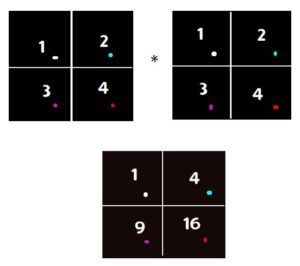# Difference between NumPy.dot() and ‘*’ operation in Python

• Last Updated : 09 Jul, 2021

In Python if we have two numpy arrays which are often referred as a vector. The ‘*’ operator and numpy.dot() work differently on them. It’s important to know especially when you are dealing with data science or competitive programming problem.

### Working of ‘*’ operator

‘*’ operation caries out element-wise multiplication on array elements. The element at a[i][j] is multiplied with b[i][j] .This happens for all elements of array.
Example:

```Let the two 2D array are v1 and v2:-
v1 = [[1, 2], [3, 4]]
v2 = [[1, 2], [3, 4]]

Output:
[[1, 4]
[9, 16]]
From below picture it would be clear.```### Working of numpy.dot()

It carries of normal matrix multiplication . Where the condition of number of columns of first array should be equal to number of rows of second array is checked than only numpy.dot() function take place else it shows an error.
Example:

```Let the two 2D array are v1 and v2:-
v1=[[1, 2], [3, 4]]
v2=[[1, 2], [3, 4]]
Than numpy.dot(v1, v2)  gives output of :-
[[ 7 10]
[15 22]]```

Examples 1:

## Python3

 `import` `numpy as np`  `# vector v1 of dimension (2, 2)``v1 ``=` `np.array([[``1``, ``2``], [``1``, ``2``]])` `# vector v2 of dimension (2, 2)``v2 ``=` `np.array([[``1``, ``2``], [``1``, ``2``]])` `print``(``"vector multiplication"``)``print``(np.dot(v1, v2))` `print``(``"\nElementwise multiplication of two vector"``)``print``(v1 ``*` `v2)`

```Output :
vector multiplication
[[3 6]
[3 6]]

Elementwise multiplication of two vector
[[1 4]
[1 4]]```

Examples 2:

## Python3

 `import` `numpy as np`  `v1 ``=` `np.array([[``1``, ``2``, ``3``], [``1``, ``2``, ``3``], [``1``, ``2``, ``3``]])` `v2 ``=` `np.array([[[``1``, ``2``, ``3``], [``1``, ``2``, ``3``], [``1``, ``2``, ``3``]]])` `print``(``"vector multiplication"``)``print``(np.dot(v1, v2))` `print``(``"\nElementwise multiplication of two vector"``)``print``(v1 ``*` `v2)`

```Output :
vector multiplication
[[ 6 12 18]
[ 6 12 18]
[ 6 12 18]]

Elementwise multiplication of two vector
[[1 4 9]
[1 4 9]
[1 4 9]]```

My Personal Notes arrow_drop_up